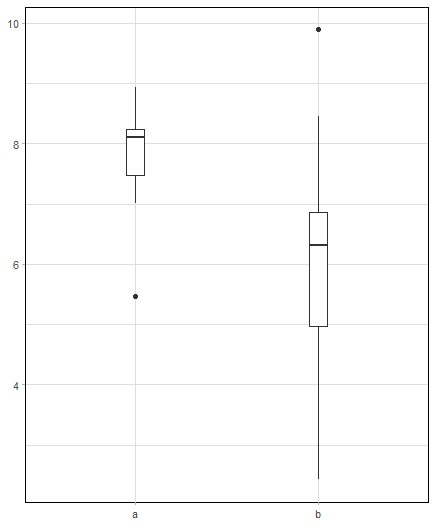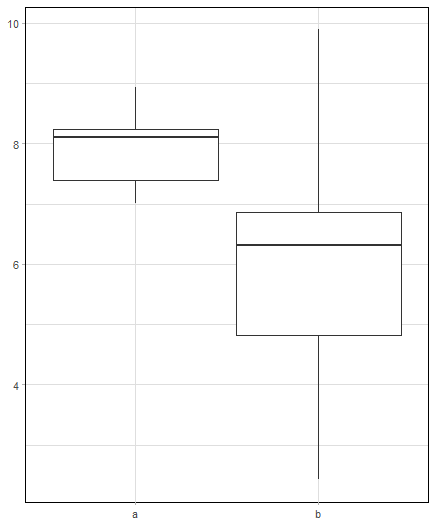Skip to content
{{ message }}

# tidyverse / ggplot2

Have a question about this project? Sign up for a free GitHub account to open an issue and contact its maintainers and the community.

By clicking “Sign up for GitHub”, you agree to our terms of service and privacy statement. We’ll occasionally send you account related emails.

Already on GitHub? Sign in to your account

# geom_boxplot with stat="identity" cannot recognize width parameter #2893

Closed
opened this issue Sep 17, 2018 · 3 comments
Closed

# geom_boxplot with stat="identity" cannot recognize width parameter#2893

opened this issue Sep 17, 2018 · 3 comments

## Comments

###artidata commented Sep 17, 2018 • edited

 These 2 plots should be the same: ```set.seed(240193) df1 <- data.frame(person=rep(c("a","b"),each = 10), score=c(rnorm(10,8,1),rnorm(10,6,1.5))) ggplot(df1)+ geom_boxplot(aes(x=person,y=score),width=0.1)``````df2 <- cbind(person = c("a","b"), as.data.frame(matrix(c(boxplot.stats(df1[1:10,2])\$stats, boxplot.stats(df1[11:20,2])\$stats),nrow=2,byrow=T))) ggplot(df2)+ geom_boxplot(aes(x = person, ymin = V1, lower = V2, middle = V3, upper = V4, ymax = V5), stat= "identity",width=0.1)```Instead I got this message = "Warning: Ignoring unknown parameters: width" The text was updated successfully, but these errors were encountered:

###tjebo commented Sep 18, 2018

 Curiously, I found the same issue yesterday just a few hours later... https://stackoverflow.com/questions/52376663/width-parameter-and-stat-identity-in-geom-boxplot?noredirect=1&lq=1 Maurits Evers (@mevers ?) has found that the width changes 'correctly', but with warning, when putting the width parameter into the aes-call
added a commit to clauswilke/ggplot2 that referenced this issue Sep 18, 2018
``` define "width" parameter for geom_boxplot. Closes tidyverse#2893. ```
``` 5ec06a5 ```

###clauswilke commented Sep 18, 2018

 Yes, `geom_boxplot()` is not properly declaring the `width` parameter. I just made a PR. ```library(ggplot2) set.seed(240193) df1 <- data.frame( person = rep(c("a", "b"), each = 10), score = c(rnorm(10, 8, 1), rnorm(10, 6, 1.5)) ) ggplot(df1) + geom_boxplot(aes(x = person, y = score), width = 0.1)``````df2 <- cbind( person = c("a", "b"), as.data.frame(matrix(c( boxplot.stats(df1[1:10, 2])\$stats, boxplot.stats(df1[11:20, 2])\$stats ), nrow = 2, byrow = T)) ) ggplot(df2) + geom_boxplot(aes( x = person, ymin = V1, lower = V2, middle = V3, upper = V4, ymax = V5 ), stat = "identity", width = 0.1 )```Created on 2018-09-18 by the reprex package (v0.2.0).
added a commit that referenced this issue Sep 19, 2018
``` define "width" parameter for geom_boxplot. Closes #2893. (#2898) ```
``` daddf74 ```

###lock bot commented Mar 18, 2019

 This old issue has been automatically locked. If you believe you have found a related problem, please file a new issue (with reprex) and link to this issue. https://reprex.tidyverse.org/
to subscribe to this conversation on GitHub. Already have an account? Sign in.
Projects
None yet
Linked pull requests

Successfully merging a pull request may close this issue.